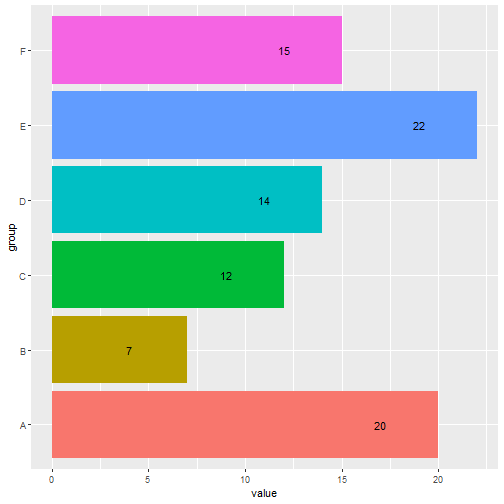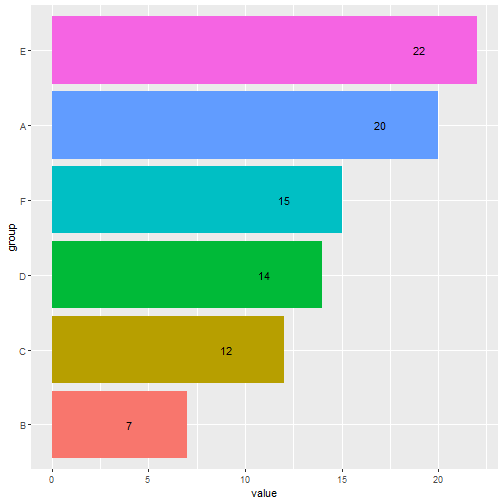# 📜 Reorder a Factor Variable in R Based on Value

##### March 14, 2022

In some situations we may want to rearrange factors based on the value of that factor. Examine the following dataset, and imagine that the group letter had no inherent meaning.

library(tidyverse)
dats
#> # A tibble: 6 x 2
#>   group value
#>   <fct> <int>
#> 1 A        20
#> 2 B         7
#> 3 C        12
#> 4 D        14
#> 5 E        22
#> 6 F        15


The issue with this is when we plot, the order of geoms on the x-axis follows this arbitrary order.

ggplot(dats, aes(x = group, y = value, fill = group)) +
geom_col() +
geom_text(aes(label = value, y = value - 3)) +
coord_flip() +
guides(fill = "none")It can sometimes be far more useful to order elements in terms of their size. To do this, you can use the fct_reorder() from the {forcats} package.

dats_forcats = dats %>%
arrange(desc(value)) %>%
mutate(group = forcats::fct_reorder(group,value))

ggplot(dats_forcats, aes(x = group, y = value, fill = group)) +
geom_col() +
geom_text(aes(label = value, y = value - 3)) +
coord_flip() +
guides(fill = "none")And there you have it. Of course, you may need to use summarize or a similar function, possibly followed by a join, if you have more complex or sophisticated criteria to arrange by. See also ??forcats::fct_reorder for more information, which shows how you can write a custom function for reordering.